25 de Mar de 2023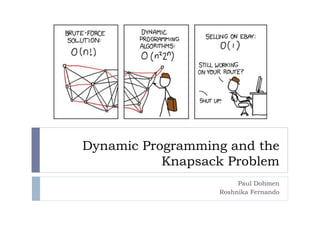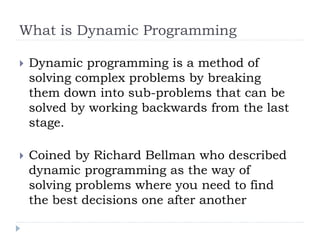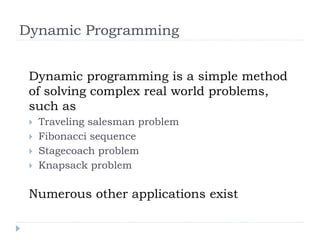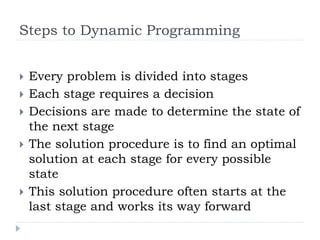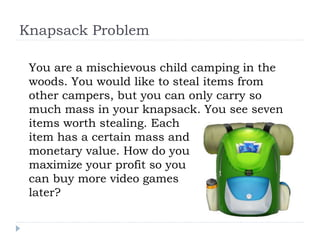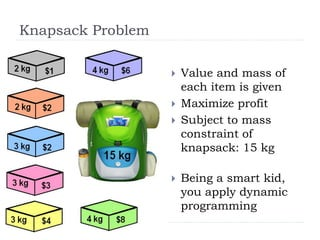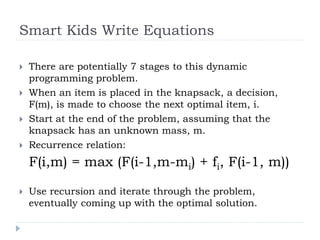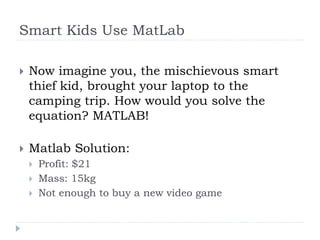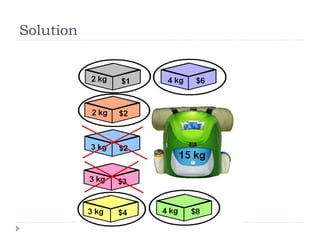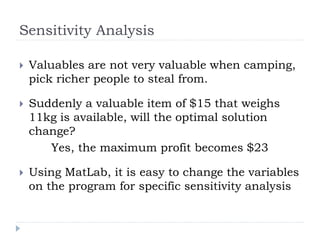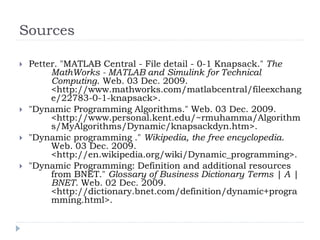1 de 12

1. Dynamic Programming and the Knapsack Problem Paul Dohmen Roshnika Fernando
2. What is Dynamic Programming  Dynamic programming is a method of solving complex problems by breaking them down into sub-problems that can be solved by working backwards from the last stage.  Coined by Richard Bellman who described dynamic programming as the way of solving problems where you need to find the best decisions one after another
3. Dynamic Programming Dynamic programming is a simple method of solving complex real world problems, such as  Traveling salesman problem  Fibonacci sequence  Stagecoach problem  Knapsack problem Numerous other applications exist
4. Steps to Dynamic Programming  Every problem is divided into stages  Each stage requires a decision  Decisions are made to determine the state of the next stage  The solution procedure is to find an optimal solution at each stage for every possible state  This solution procedure often starts at the last stage and works its way forward
5. Knapsack Problem You are a mischievous child camping in the woods. You would like to steal items from other campers, but you can only carry so much mass in your knapsack. You see seven items worth stealing. Each item has a certain mass and monetary value. How do you maximize your profit so you can buy more video games later?
6. Knapsack Problem  Value and mass of each item is given  Maximize profit  Subject to mass constraint of knapsack: 15 kg  Being a smart kid, you apply dynamic programming
7. Smart Kids Write Equations  There are potentially 7 stages to this dynamic programming problem.  When an item is placed in the knapsack, a decision, F(m), is made to choose the next optimal item, i.  Start at the end of the problem, assuming that the knapsack has an unknown mass, m.  Recurrence relation: F(i,m) = max (F(i-1,m-mi) + fi, F(i-1, m))  Use recursion and iterate through the problem, eventually coming up with the optimal solution.
8. Smart Kids Use MatLab  Now imagine you, the mischievous smart thief kid, brought your laptop to the camping trip. How would you solve the equation? MATLAB!  Matlab Solution:  Profit: \$21  Mass: 15kg  Not enough to buy a new video game
9. Solution
10. Sensitivity Analysis  Valuables are not very valuable when camping, pick richer people to steal from.  Suddenly a valuable item of \$15 that weighs 11kg is available, will the optimal solution change? Yes, the maximum profit becomes \$23  Using MatLab, it is easy to change the variables on the program for specific sensitivity analysis
11. Questions?
12. Sources  Petter. "MATLAB Central - File detail - 0-1 Knapsack." The MathWorks - MATLAB and Simulink for Technical Computing. Web. 03 Dec. 2009. <http://www.mathworks.com/matlabcentral/fileexchang e/22783-0-1-knapsack>.  "Dynamic Programming Algorithms." Web. 03 Dec. 2009. <http://www.personal.kent.edu/~rmuhamma/Algorithm s/MyAlgorithms/Dynamic/knapsackdyn.htm>.  "Dynamic programming ." Wikipedia, the free encyclopedia. Web. 03 Dec. 2009. <http://en.wikipedia.org/wiki/Dynamic_programming>.  "Dynamic Programming: Definition and additional resources from BNET." Glossary of Business Dictionary Terms | A | BNET. Web. 02 Dec. 2009. <http://dictionary.bnet.com/definition/dynamic+progra mming.html>.